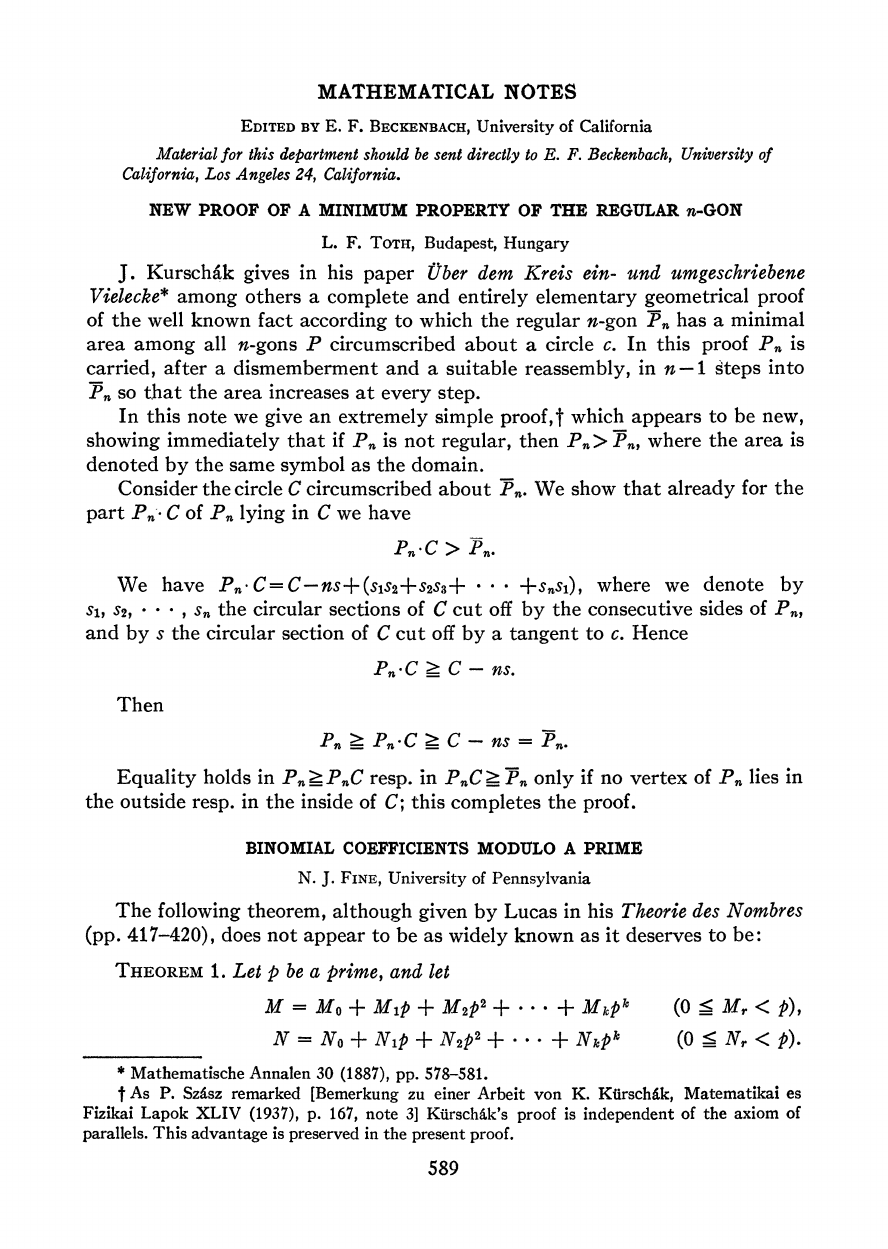László Fejes Tóth was a Hungarian mathematician who specialized in ...
In elementary geometry, a polygon is a plane figure that is bounded...
Toth's proof starts with a construction of the circumcircle $C$ of ...
Now for $P_n$ we can have vertices lying inside or outside $C$. ...
Since $P_n$ might expand outside of $C$ it is obvious that $P_n \ge...MATHEMATICAL NOTES EDITED BY E. F. BECKENBACIE, University of California Material for this department should be sent directly to E. F. Beckenbach, University of California, Los Angeles 24, California. NEW PROOF OF A MINIMUM PROPERTY OF THE REGULAR n-GON L. F. ToTH, Budapest, Hungary J. Kurschik gives in his paper Ober dem Kreis ein- und urmgeschriebene Vielecke* among others a complete and entirely elementary geometrical proof of the well known fact according to which the regular n-gon Pn has a minimal area among all n-gons P circumscribed about a circle c. In this proof P. is carried, after a dismemberment and a suitable reassembly, in n -I steps into Pn so that the area increases at every step. In this note we give an extremely simple proof,t which appears to be new, showing immediately that if Pn is not regular, then P, ,,, where the area is denoted by the same symbol as the domain. Consider the circle C circumscribed about P. We show that already for the part Pn- C of P. lying in C we have Pn-C > Pn. We have Pn C=C-ns+(S1S2+S2s3+ ** +SnSI), where we denote by S, S2, S* * , sn the circular sections of C cut off by the consecutive sides of Pn, and by s the circular section of C cut off by a tangent to c. Hence Pn*C _ C - nS. Then P7 >! Pn C > C - ns =Pn. Equality holds in P. r! P.C resp. in PnC ? Pn only if no vertex of Pn lies in the outside resp. in the inside of C; this completes the proof. BINOMIAL COEFFICIENTS MODULO A PRIME N. J. FINE, University of Pennsylvania The following theorem, although given by Lucas in his Theorie des Nombres (pp. 417-420), does not appear to be as widely known as it deserves to be: THEOREM 1. Let p be a prime, and let M = MO + MIP + M2p2 + * . + Mkpk (O < Mr < P), No + Np + N2p2+ ** +Nkpk (O 5 Nr < P). * Mathematische Annalen 30 (1887), pp. 578-581. t As P. Sz6sz remarked [Bemerkung zu einer Arbeit von K. Ktirschik, Matematikai es Fizikai Lapok XLIV (1937), p. 167, note 3] Kurschik's proof is independent of the axiom of parallels. This advantage is preserved in the present proof. 589 This content downloaded from 128.235.251.160 on Mon, 19 Jan 2015 14:44:41 PM All use subject to JSTOR Terms and Conditions In this proof regular polygon seems to denote a convex regular polygon. ($\overline{P_n} ≠ C - ns$for a regular star$n$-gon) You are right Neven. @Ning no. Does this mean that any polygon can be constructed from a regular polygon by extending/shortening of one or more of its sides? László Fejes Tóth was a Hungarian mathematician who specialized in geometry. He proved that a lattice pattern is the most efficient way to pack centrally symmetric convex sets on the Euclidean plane. He also investigated the sphere packing problem. Tóth worked extensively with H.S.M. Coxeter and Paul Erdős, with whom he laid the foundations of discrete geometry. ![](https://upload.wikimedia.org/wikipedia/commons/a/a2/L%C3%A1szl%C3%B3_Fejes_T%C3%B3th-1958-TableTennis.png) Toth's proof starts with a construction of the circumcircle$C$of the regular n-gon$\overline{P}_n$that circumscribes$c$. ![](http://i.imgur.com/Hcr6PS1.png?1) As can be seen in the image above, all the sides of$\overline{P}_n$cut the same circular section$s$of$C$because each side is the same distance from the center$O$. As a consequence, the area of$\overline{P}_n=C-ns$. In elementary geometry, a polygon is a plane figure that is bounded by a finite chain of straight line segments closing in a loop. Here are a few examples of polygons. ![](https://upload.wikimedia.org/wikipedia/commons/1/1f/Assorted_polygons.svg) A regular polygon is a polygon where all angles are equal in measure and all sides have the same length. Regular polygons may be convex or star. ![](https://upload.wikimedia.org/wikipedia/commons/thumb/7/76/Regular_star_polygons.svg/512px-Regular_star_polygons.svg.png) Now for$P_n$we can have vertices lying inside or outside$C$. ![](http://i.imgur.com/LBLaAnC.png?1) Since all sides of$P_n$and$\overline{P}_n$are tangents to$c$, each side of$P_n$that cuts all the way through$C$will cut from it a chunk of area$s$. For the vertices that occur in the ring between$c$and$C$we will extend both sides so that they cut all the way through$C$as can be seen in the following image (the area of these chunks will be$s$for the reason discussed above). We will also name the circular sections cut off by the consecutive sides of$P_ns_1,s_2,...,s_n$. ![](http://i.imgur.com/Vw6aZ05.png?1) To calculate$P_n \cdot C$, the area of$P_n$lying in$C$we need to subtract from$C$the areas cut by the sides of$P_n$. For the sides meeting at a vertex outside of$C$each side cuts a full$s$from$C$. For the sides meeting at a vertex in the ring between$c$and$C$we have 2 segments$s_i$and$s_{i+1}$(each with area$s$) which overlap. If we subtract and area$s$for each of$s_i$we would be taking away their intersection$s_is_{i+1}$twice, and so we need to correct this by adding the area of the intersection$s_is_{i+1}$for each vertex. Finally $$P_n \cdot C = C-ns+(s_1s_2 + s_2s_3 + ... + s_ns_1)$$ Note that if the vertex lies exactly on$C$,$s_is_{i+1}=0$for that vertex. Since$P_n$might expand outside of$C$it is obvious that$P_n \geq P_n \cdot C = C-ns+(s_1s_2 + s_2s_3 + ... + s_ns_1)$. Also since the areas of the intersections$s_is_{i+1}$are not negative,$(s_1s_2 + s_2s_3 + ... + s_ns_1)$is not negative as well and so$P_n \geq P_n \cdot C \geq C-ns = \overline{P}_n$. We finally proved that the regular n-gon$\overline{P}_n$has a minimal area among all n-gons$P_n$! Note that if$P_n$has any vertex outside$C$we have$P_n > P_n \cdot C$and if$P_n$has a vertex in the ring between$c$and$C$we have$(s_1s_2 + s_2s_3 + ... + s_ns_1)>0$and thus $$P_n \cdot C > C-ns = \overline{P}_n$$ The only case when the two areas are equal$P_n=\overline{P}_n $is when all the vertices of$P_n$are on C, which means that$P_n\$ is also a regular n-gon.